# Uniswap V3: Liquidity providing 101

Hello there, no time for the pleasantries, let’s talk Uniswap V3 math!

We’re going to deep dive into how liquidity provision works in Uniswap v3 and how you can align the portfolio of 2 tokens so that your LP position yields the maximum fees while reducing IL to the minimum. Expect a lot of formulas and numbers.

# Uniswap v2 recap: a trader’s perspective

Uniswap v2 is an automated market maker that allows:

• Traders to swap one asset for another;
• Liquidity providers (LPs) to provide liquidity and earn trading fees.

Each pool has 2 tokens: X and Y. If the pool holds xₚ tokens of X and yₚ tokens of Y (also called pool reserves), then pool liquidity L is defined by:

The current ratio of tokens in the pool defines the current swap price p:

If a trader wants to swap y tokens of Y, they deposit y tokens into the pool and receive x tokens of X back. x is defined by the pool using the following equation:

Here ϕ is a pool fee. For Uni v2, it’s 0.3%.

Now assume for a moment that ϕ = 0, and let’s see what happens if the current price p = 1, pool reserves xₚ = 1, yₚ = 1, and a trader wants to swap y = 1 units of Y token.

The trader will receive back x = 0.5 tokens of X. The pool reserves and price will update to xₚ = 0.5, yₚ = 2, p = 4.

We would expect x = 1 at current price p = 1 but the actual x amount is subject to loss known as slippage (sₗ = 0.5):

The good news is sₗ → 0 as pool liquidity L grows or swap amount decreases, i.e., for small enough swap amounts you exchange at price p fewer fees.

# Uniswap v2 recap: a liquidity provider’s perspective

Now let’s see what happens if p = 4, pool reserves xₚ = 0.5, yₚ = 2, L = 1 and a liquidity provider wants to put x = 0.25 tokens of X and y = 1 tokens of Y.

In this case, the new reserves for the pool will be xₚ = 0.75, yₚ = 3 and = xₚ · yₚ = 2.25, so L = 1.5 and liquidity provider receives ΔL = 0.5 liquidity in the form of Uni V2 lp tokens. Now on each trade, a liquidity provider will receive ΔL / L = 1 / 3 share of fees.

In this example we purposefully used x = 0.25 and y = 1 as liquidity provider’s investment so that y / x = 4 = p.

What happens if y / x ≠ p? In this case, some of the tokens Δx or Δy are returned to the liquidity provider so that the leftover ratio (y-Δy)/ x = p or y / (x-Δx) = p. If the liquidity provider wants to utilize their tokens to the full and receive max liquidity, they first must trade their tokens so that y / x = p and then put them into the pool.

For Uni v2, it’s quite straightforward to align your token portfolio to get the maximum liquidity. What about Uni v3? It turns out things are getting way more complex there.

# Uniswap v3: single position

In May 2021 Uniswap team launched v3. On Uni v3, you can put liquidity on any price interval [pᵃ, pᵇ]. As long as the price is in the range [pᵃ, pᵇ], you have liquidity L and earn fees. When the price is out of range, you don’t earn any fees until the price is back in the range.

Let’s see how it works in action. First, let’s consider the pool with only one open position with the price interval [pᵃ, pᵇ] = [0.25, 4]. Current pool reserves are xₚ = yₚ = 0.5, the price is p = 1. In this case, as swaps are happening and the price is moving, we observe the following pool behavior:

Here the green curve is the actual token reserves that are used for swaps (real liquidity curve), and the red curve is a virtual liquidity curve emulating as if a user is swapping on Uni v2.

As long as the price is in the range [0.25, 4], the pool behavior is exactly the same as if the pool was UniV2 with a red liquidity curve. When the price is out of bounds, virtual liquidity drops to zero, real liquidity concentrates either in X or in Y token and is not used for swaps.

# Uniswap v3: multiple positions

Let’s see now what happens if there are 2 liquidity investments x₁, y₁ on [pᵃ₁, pᵇ₁] and x₂, y₂ on [pᵃ₂, pᵇ₂]. Each of these investments implies (we’ll see how in the following chapters) virtual liquidities L₁ and L₂:

As can be seen, when both intervals cover the price, both real reserves are used, and pool virtual liquidity equals the sum of liquidities. When only one interval covers the price — only its liquidity is used. When the price is out of both intervals, the pool liquidity is zero (or you don’t earn any fees).

This gives Uni v3 a unique feature — a piecewise liquidity function. As the price moves along the virtual curve, the liquidity value changes by some ΔL at certain price points (that are bounds of liquidity positions). You can see such jumps happening at prices pᵃ₁ and pᵇ₁ in Figure 3.

# Ticks and tick spacing

The actual life of Uniswap v3 is a bit harder than shown in the figures above. In real Uni v3, you cannot put your liquidity on an arbitrary price interval. Instead, there are so-called ticks that form a discrete grid over the price range. Ticks are defined by the formula (i is an integer):

For each pool, there’s also a notion of tick spacing. Tick spacing is yet another grid on top of ticks that limits the ticks in which you can put liquidity. E.g., for 0.3%-fee pool tick spacing is 60 so that you can put liquidity only in each 60ᵗʰ tick, e.g., 0, -60, 60, 120, -120, … The figure below shows tick spacing ticks (orange) and ticks (black)

Because your liquidity price interval bounds can only be the tick spacing ticks, the liquidity inside any space tick interval is constant and can only change when the price crosses the tick spacing tick.

So we have a piecewise liquidity function with possible jumps happening at tick spacing ticks (similar to Figure 2).

# Liquidity value for a position

Let’s see how liquidity L is calculated, given initial tokens x and y, price interval [pᵃ, pᵇ], and current price p.

As you can see from these equations, if the x and y tokens are not in the right proportion (Lx≠Ly), some tokens are returned to the liquidity provider. This is similar to the behavior we observed in Uni v2.

But for Uni v3, it’s more complex since we have the piecewise liquidity function with jumps at tick spacing ticks. In the following chapter, we’ll show how to put tokens in the most effective way on Uni v3.

# Effective liquidity providing

If we have a portfolio of x tokens of X and y tokens of Y and we want to provide liquidity to price range [pᵃ, pᵇ] how many tokens X or Y should we swap to get the maximum liquidity out of it?

To answer this question, let’s denote R = y / x — the ratio of tokens in our portfolio and rᵃᵇ(p) — the optimal token ratio such that Lx = Ly. From the formulas for Lx and Ly we can derive:

So our goal is to make R = rᵃᵇ.

However, the task is a bit more complex than just aligning x and y to the specified ratio rᵃᵇ. As we start swapping x for y or vice versa, the pool price p starts changing and so does rᵃᵇ. This behavior is shown in the figure below:

Yet another layer of complexity is as the price p is changing and crossing a tick spacing tick pool liquidity L is changing as well! To solve this problem, let’s first understand how the ratio R evolves as we swap token Y for token X given the liquidity L is constant.

From the equations L²=xy and p = y / x it’s easy to derive:

So if we make a swap of Y for X, subtract fees from Y and the price after swap settles at p₁ (p₁ > p₀):

If the direction is X for Y then p₁ < p₀ and we have:

The next question is:

At what ratio R does the pool price cross the tick and liquidity changes?

If we denote R+ as the ratio at which the upper tick is crossed and R- the ratio for the lower tick, p₀ is the initial price and p-, p+ are respective prices at ticks, L — current liquidity at the tick spacing interval, then we have:

Finally, we are ready to solve the problem.

First, we need to answer 2 questions:

1. Is R > rᵃᵇ(p₀)? If yes, we need to swap Y for X, otherwise — X for Y.
2. As we swap — will the pool price ever cross a tick spacing tick? If no — we can solve the problem right away. If yes — we need to adjust our values as if we swapped all the way to the tick and then repeat our algorithm on the new tick with the new liquidity.

The answers to these questions bring us to 4 different cases:

1. Swapping Y for X inside one tick interval: R > rᵃᵇ(p₀), R+ ≤ rᵃᵇ(p+)
2. Swapping X for Y inside one tick interval: R < rᵃᵇ(p₀), R- ≥ rᵃᵇ(p-)
3. Swapping Y for X in different tick intervals: R > rᵃᵇ(p₀), R+ > rᵃᵇ(p+)
4. Swapping X for Y in different tick intervals: R < rᵃᵇ(p₀), R- < rᵃᵇ(p-)

Case 1: Swapping Y for X inside one tick interval: R > rᵃᵇ(p₀), R+ ≤ rᵃᵇ(p+)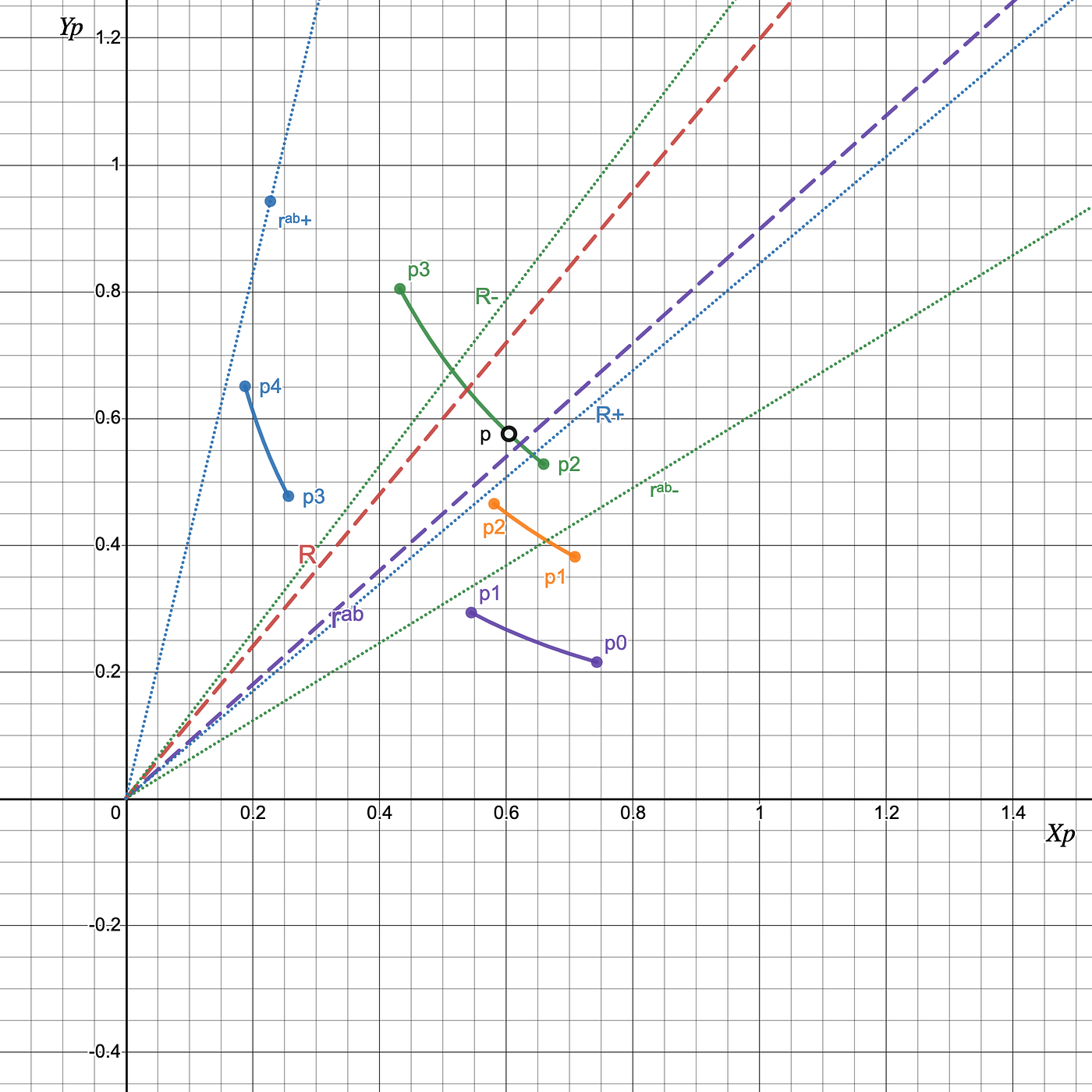Figure 7: Swapping Y for X inside one tick interval. As we swap from Y to X the price is growing to p₃, R is decreasing to R+, while rᵃᵇ is increasing to rᵃᵇ+. Since initially R > rᵃᵇ and R+ ≤ rᵃᵇ+ it is guaranteed that R = r is inside the tick interval.

If we swap y for x and the price after the swap settles at p₁ all we need is to make sure Ryx(p₁) = rᵃᵇ(p₁):

If we assume z = √p₁ and rearrange the terms of the equation we’ll get a quadratic equation:

Thus we can solve it and find p₁:

And the amount of token Y to swap is:

Case 2: Swapping X for Y inside one tick interval: R < rᵃᵇ(p₀), R- ≥ rᵃᵇ(p-)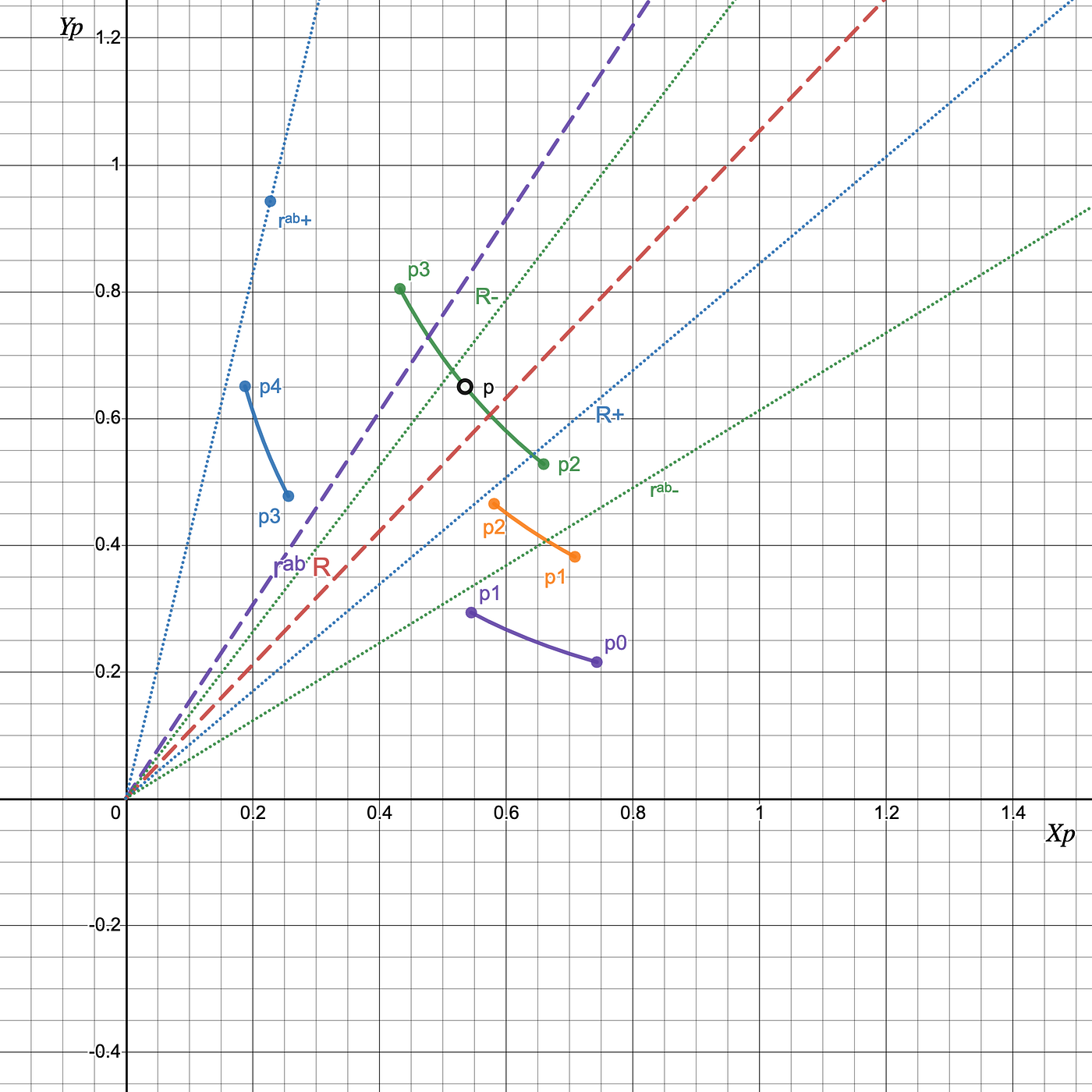Figure 8: Swapping X for Y inside one tick interval. As we swap from X to Y the price is decreasing to p₂, R is growing to R-, while rᵃᵇ is decreasing to rᵃᵇ-. Since initially R < rᵃᵇ and R- ≥ rᵃᵇ- it is guaranteed that R = r is inside the tick interval.

This case is very similar to case 1, except we swap X for Y and thus we need to make sure Rxy(p₁) = rᵃᵇ(p₁):

Similarly to case 1, we can find:

And the amount of token X to swap is:

Case 3: Swapping Y for X in different tick intervals: R > rᵃᵇ(p₀), R+ > rᵃᵇ(p+)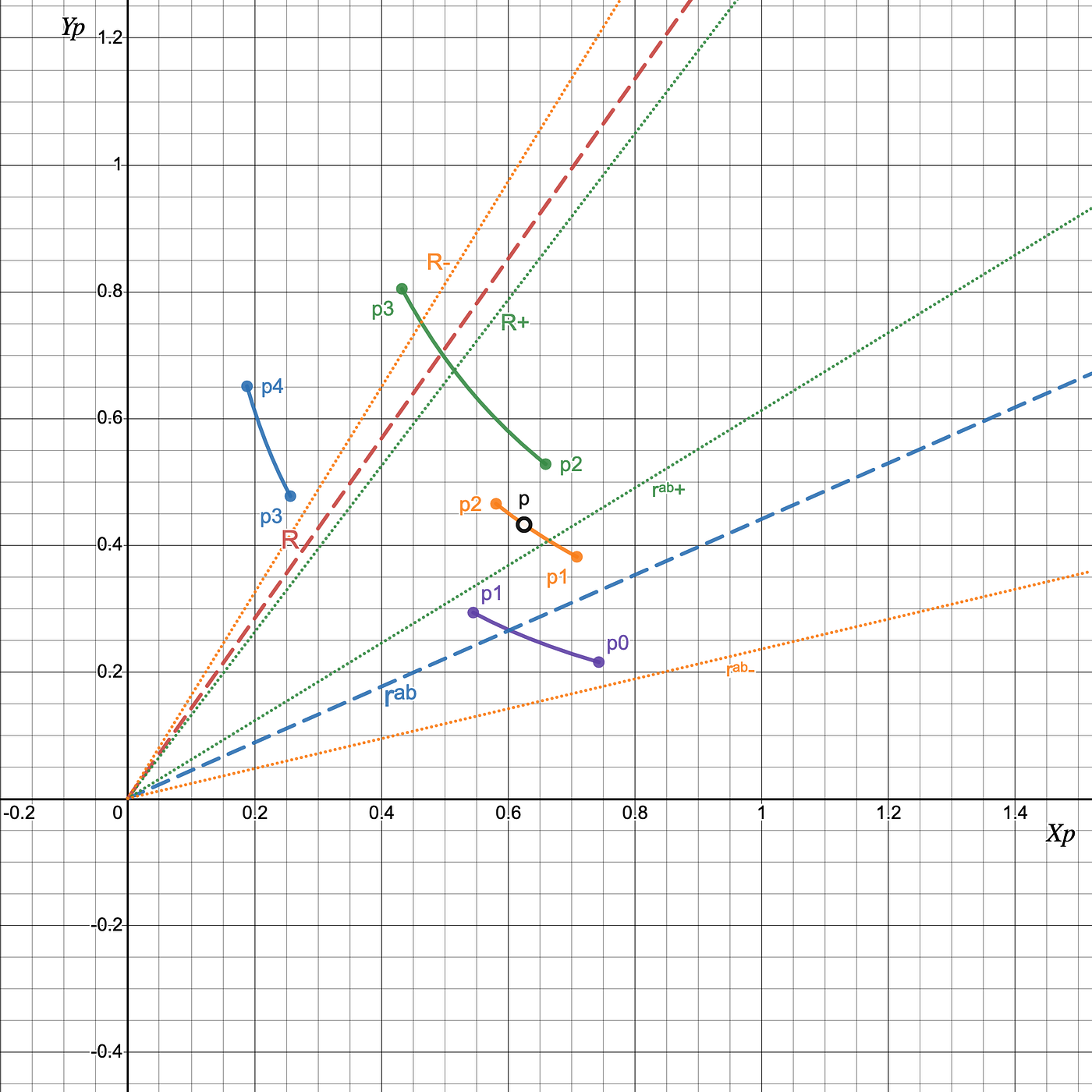Figure 9: Swapping Y for X in different tick intervals. As we swap from Y to X the price is growing to p₂ and then to p₃, R is decreasing to R+, while rᵃᵇ is increasing to rᵃᵇ+. Since initially R > rᵃᵇ and R+ > rᵃᵇ+ it is guaranteed that the price will cross tick spacing tick while we swap to R = rᵃᵇ

In this case, we swap Y for X until R = R+ and so p = p+ and we’re in the new tick interval with the new liquidity. We remember how many tokens of Y we swapped to add them to the final numbers later. Then we start the algorithm all over and repeat until we hit case 1. That is we redefine:

Case 4: Swapping X for Y in different tick intervals: R < rᵃᵇ(p₀), R- < rᵃᵇ(p-)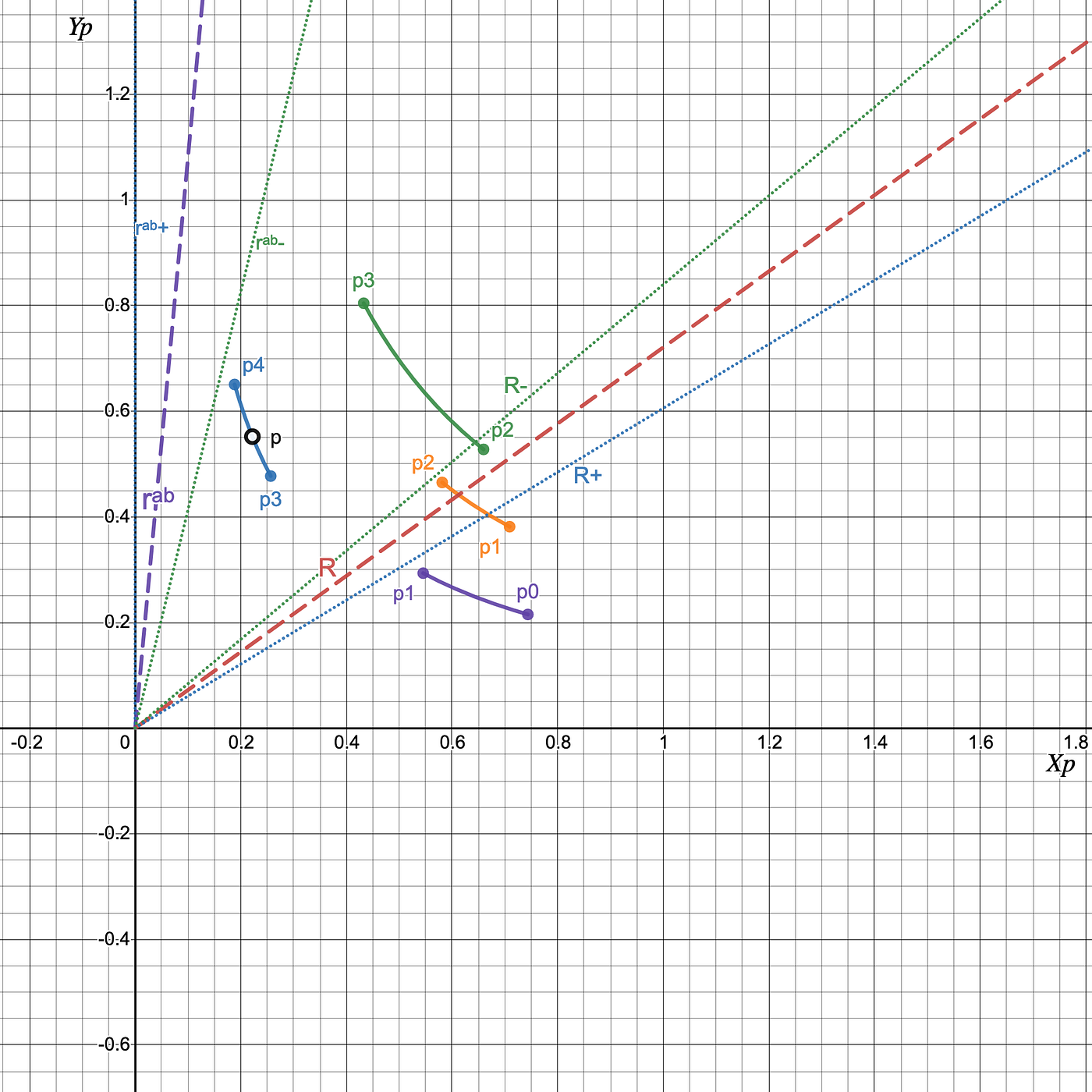Figure 10: Swapping X for Y in different tick intervals. As we swap from X to Y the price is decreasing to p₃ and then to p₂, R is increasing to R-, while rᵃᵇ is decreasing to rᵃᵇ-. Since initially R < rᵃᵇ and R- < rᵃᵇ- it is guaranteed that the price will cross tick spacing tick while we swap to R = rᵃᵇ

Exactly similar to case 3 except we swap X for Y until R = R- and repeat until we hit case 2. We redefine:

# Conclusion

Supplying liquidity in the right proportion in Uni v3 is a very complex task. You need to consider a lot of factors like different liquidity values inside tick intervals. The algorithm above describes how you can use the pool data to calculate the number of tokens you need to swap to get the highest liquidity.

The charts for figures are available here:

https://www.desmos.com/calculator/rqbrnapxbj

https://www.desmos.com/calculator/ys7kcfgjxe

https://www.desmos.com/calculator/ehdwkbtu7z

https://www.desmos.com/calculator/huzzwffze9

https://www.desmos.com/calculator/t9u9x8xgcy

In future articles, we plan to discuss other interesting aspects of Uni v3 like impermanent loss, multiposition portfolios, strategy risks, etc.

# Who we are

Mellow Protocol is exploring the space of LP-ing and market-making on AMMs. You can also check our initial research paper here. The goal of Mellow is to build a robust ecosystem of tools for eliminating market inefficiencies and generating outcome for users.

We don’t see this just as a product, but as an evolution of what complex math can bring to the DeFi space. Similar to how Uniswap and Curve innovated user trading experience, we believe LP optimization is also pushing forward the boundaries of what was possible in tradfi.

In the next articles, we’re going to discuss more ideas on how to implement proper rebalancing strategies and will move forward to broader topics in DeFi.

Stay tuned!

Follow Mellow on Twitter to be in the loop with our research, and join the discussion in Discord.

--

--

--

## More from Mellow Protocol

Exploring the depths of math on LPing, AMMs and trading strategies.

Love podcasts or audiobooks? Learn on the go with our new app.

## Create your crypto platform with DApps Development to top the blockchain market## FalconSwap alpha Launch## The Rise of Hybrid Blockchain Networks## What a launch!## Let’s talk about our Crowdsale & KYC## DOC-related Airdrop Reward Distribution Announcement [EN/KR]## Where are the GApps in Bitcoin and Ethereum?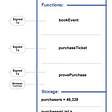## Mellow Protocol

Exploring the depths of math on LPing, AMMs and trading strategies.

## Uniswap v3 liquidity formula explained## Gamma transforms: How to hedge squeeth using Uni V3## A Brief History of Uniswap and Its Math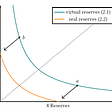## Expected Impermanent Loss in Uniswap V2 & V3